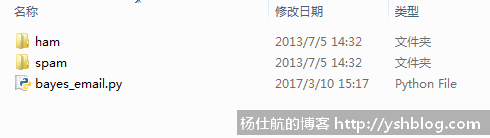### 机器学习08：朴素贝叶斯应用(词袋模式)

• 发布时间：2017年3月10日 16:16
• 作者：杨仕航

```def set_of_words_to_bag(vocab_list, input_set):
'''对比句子向量和词汇表，找到对应出现在词汇表的位置以及个数'''
return map(lambda x:input_set.count(x), vocab_list)```

bayes_email.py文件中核心的朴素贝叶斯算法的代码无需改变，思路一致。

《机器学习实战》已经给我们提供相关数据，打开如下链接，下载email.zip文件。

https://github.com/pbharrin/machinelearninginaction/tree/master/Ch04ham和spam文件夹中各有25个txt文件。每个文件都代表一封邮件的内容。邮件内容是正常的句子，所以我们不能只是使用空格拆分单词，还要考虑标点符号等等。

```#coding:utf-8
import os
import re

#拆分邮件
def text_parse(text):
#使用非字母和非数字的字符拆分
words = re.split(r'\W*', text)

#保留长度大于2的单词
return [x.lower() for x in words if len(x)>2]```

```def create_dataset():
#遍历ham和spam文件夹，获取邮件内容列表
folders = [u'ham', u'spam']
posting_list = []
class_vect = []

for i, folder in enumerate(folders):
for f in os.listdir(folder):
#判断文件是否为txt文件
if os.path.splitext(f)[-1] != '.txt':
continue

#合并路径，读取文件内容
f_path = os.path.join(folder, f)
with open(f_path, 'r') as txt:
#新增文本拆分结果
#新增该文本所属类别。ham为0,spam为1
class_vect.append(i)

return posting_list, class_vect```（不要问朴素贝叶斯是什么，朴素贝叶斯其他代码在哪里。看前面两篇博文）

```#测试方法
def testing_nb():
#创建训练集
posting_list, class_vect = create_dataset()

#获取词汇表
vocab_list = create_vocab_list(posting_list)

#句子单词数字化
posting_vects = []
for posting in posting_list:
posting_vect = set_of_words_to_bag(vocab_list, posting)
posting_vects.append(posting_vect)

#获得相关概率
p_vect, p_class = train_nb0(np.array(posting_vects), np.array(class_vect))

#以上是通用代码，下面是分类器测试
#测试分类器
#遍历ham和spam文件夹，获取邮件内容列表
folders = [u'ham', u'spam']
err_num = 0
all_num = 0

for i, folder in enumerate(folders):
for f in os.listdir(folder):
#判断文件是否为txt文件
if os.path.splitext(f)[-1] != '.txt':
continue

#合并路径，读取文件内容
f_path = os.path.join(folder, f)
with open(f_path, 'r') as txt:
#文本拆分
vect_test = set_of_words_to_bag(vocab_list, words)

#用朴素贝叶斯判断该邮件内容类别
class_type, _ = classify_nb(vect_test, p_vect, p_class)

#判断结果是否正确
if class_type != i:
err_num += 1
all_num += 1
#返回错误率
return err_num / float(all_num)```（错误率竟然比《机器学习实战》书中的错误率还要低）

```#coding:utf-8
#词袋模式，以句子为单位，体现单词出现次数
import numpy as np
import os
import re

#拆分邮件
def text_parse(text):
#使用非字母和非数字的字符拆分
words = re.split(r'\W*', text)

#保留长度大于2的单词
return [x.lower() for x in words if len(x)>2]

def create_dataset():
#遍历ham和spam文件夹，获取邮件内容列表
folders = [u'ham', u'spam']
posting_list = []
class_vect = []

for i, folder in enumerate(folders):
for f in os.listdir(folder):
#判断文件是否为txt文件
if os.path.splitext(f)[-1] != '.txt':
continue

#合并路径，读取文件内容
f_path = os.path.join(folder, f)
with open(f_path, 'r') as txt:
#新增文本拆分结果
#新增该文本所属类别。ham为0,spam为1
class_vect.append(i)

return posting_list, class_vect

def create_vocab_list(dataset):
'''对所有句子取并集，获取词汇表'''
return list(reduce(lambda x,y:x|set(y), [set([])] + dataset))

def set_of_words_to_bag(vocab_list, input_set):
'''对比句子向量和词汇表，找到对应出现在词汇表的位置以及个数'''
return map(lambda x:input_set.count(x), vocab_list)

#训练函数（句子数字化之后的矩阵，句子的性质列表）
def train_nb0(posting_vects, train_classes):
num_train_docs = len(posting_vects) #总句子数
num_words = len(posting_vects)   #词汇表单词数

#遍历分类标签，统计对应类别的数量
p_class_num = {} #各类别句子数
p_vect_num = {}  #各类别各单词数

for i, class_type in enumerate(train_classes):
#累计每个类别的单词数（初始化每个类别单词数为1个）
p_vect_num[class_type] = p_vect_num.get(class_type, np.ones(num_words)) + posting_vects[i]

#累计每个类别的句子数
p_class_num[class_type] = p_class_num.get(class_type, 0) + 1

#计算每个类别的条件概率，对应单词数除以该类别的单词总数
p_class = {} #各类别的概率
p_vect = {}  #各类别的各单词条件概率

for class_type in train_classes:
p_vect[class_type] = p_vect_num[class_type]/np.sum(p_vect_num[class_type])
p_class[class_type] = p_class_num[class_type]/float(num_train_docs)
return p_vect, p_class

#朴素贝叶斯分类函数
def classify_nb(vect_classify, p_vect, p_class):
#计算句子属于各类别的概率
p = {}
for class_type, vect in p_vect.items():
p[class_type] = np.sum(vect_classify * np.log(vect)) + np.log(p_class[class_type])

#获取最大概率的类别
return max(p.items(), key=lambda x:x)

#测试方法
def testing_nb():
#创建训练集
posting_list, class_vect = create_dataset()

#获取词汇表
vocab_list = create_vocab_list(posting_list)

#句子单词数字化
posting_vects = []
for posting in posting_list:
posting_vect = set_of_words_to_bag(vocab_list, posting)
posting_vects.append(posting_vect)

#获得相关概率
p_vect, p_class = train_nb0(np.array(posting_vects), np.array(class_vect))

#以上是通用代码，下面是分类器测试
#测试分类器
#遍历ham和spam文件夹，获取邮件内容列表
folders = [u'ham', u'spam']
err_num = 0
all_num = 0

for i, folder in enumerate(folders):
for f in os.listdir(folder):
#判断文件是否为txt文件
if os.path.splitext(f)[-1] != '.txt':
continue

#合并路径，读取文件内容
f_path = os.path.join(folder, f)
with open(f_path, 'r') as txt:
#文本拆分
vect_test = set_of_words_to_bag(vocab_list, words)

#用朴素贝叶斯判断该邮件内容类别
class_type, _ = classify_nb(vect_test, p_vect, p_class)

#判断结果是否正确
if class_type != i:
err_num += 1
all_num += 1
#返回错误率
return err_num / float(all_num)

if __name__ == '__main__':
print(testing_nb())```